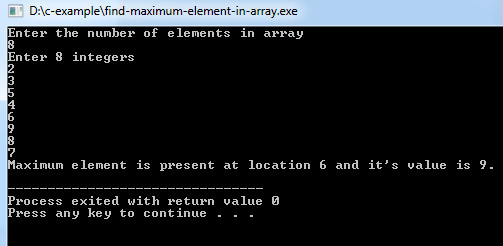## C Programming Examples Tutorial Index

This program find maximum or largest element present in an array. It also prints the location or index at which maximum element occurs in array.

Program:

``````#include <stdio.h>

int main()
{
int array, maximum, size, c, location = 1;

printf("Enter the number of elements in array\n");
scanf("%d", &size);

printf("Enter %d integers\n", size);

for (c = 0; c < size; c++)
scanf("%d", &array[c]);

maximum = array;

for (c = 1; c < size; c++)
{
if (array[c] > maximum)
{
maximum  = array[c];
location = c+1;
}
}

printf("Maximum element is present at location %d and it's value is %d.\n", location, maximum);
return 0;
}``````

Program Output:## Same C program code using pointers

Example:

``````#include <stdio.h>

int main()
{
long array, *maximum, size, c, location = 1;

printf("Enter the number of elements in array\n");
scanf("%ld", &size);

printf("Enter %ld integers\n", size);

for ( c = 0 ; c < size ; c++ )
scanf("%ld", &array[c]);

maximum  = array;
*maximum = *array;

for (c = 1; c < size; c++)
{
if (*(array+c) > *maximum)
{
*maximum = *(array+c);
location = c+1;
}
}

printf("Maximum element found at location %ld and it's value is %ld.\n", location, *maximum);
return 0;
}``````

The complexity of above code is O(n) as the time used depends on the size of input array or in other words time to find maximum increases linearly as array size grows.